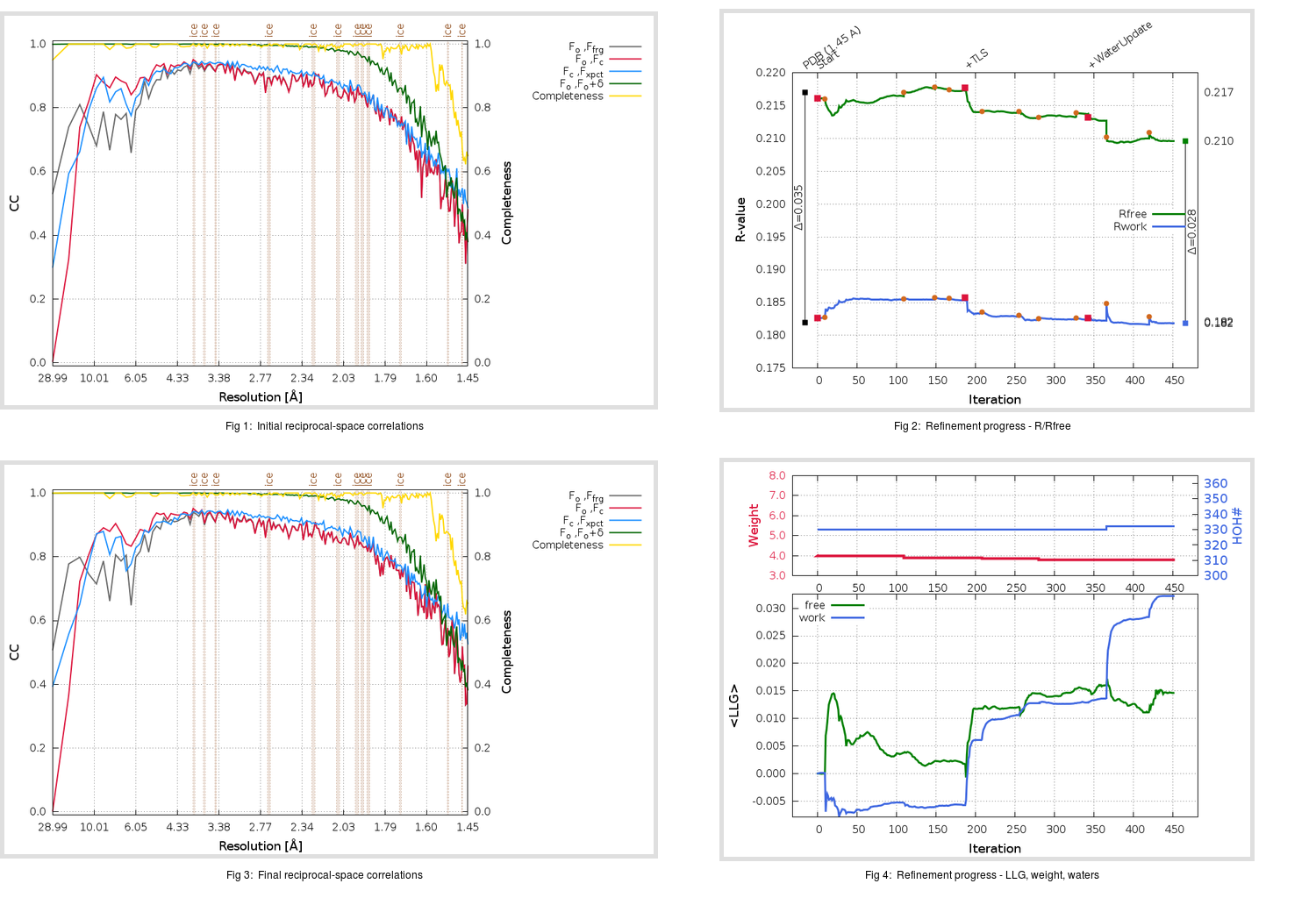Content:

```    Diffraction limits & principal axes of ellipsoid fitted to diffraction cut-off surface:
1.613         0.9325   0.0000  -0.3612       0.973 a* - 0.232 c*
1.479         0.0000   1.0000   0.0000       b*
1.439         0.3612   0.0000   0.9325       0.741 a* + 0.671 c*
```

## Deposited

` `
 Date deposited Date data collection Resolution R, Rfree 20200315 20200305 1.45 0.1810 0.2170

Molprobity (CCP4 7.0 version) summary:

```Ramachandran outliers =   0.33 %
favored =  98.34 %
Rotamer outliers      =   1.52 %
C-beta deviations     =     0
Clashscore            =   4.22
RMS(bonds)            =   0.0134
RMS(angles)           =   1.84
MolProbity score      =   1.34
Resolution            =   1.45
R-work                =   0.1810
R-free                =   0.2170
```

```Number of waters      =   330

<B> (all atoms) =   22.68 ( sd =    8.92 ) for       2731 non-hydrogen atoms
<B>   (protein) =   21.12 ( sd =    7.73 ) for       2370 non-hydrogen atoms
<B>     (water) =   33.36 ( sd =    9.27 ) for        330 non-hydrogen atoms
<B>    (others) =   28.05 ( sd =   11.48 ) for         31 non-hydrogen atoms

B min/max       (all non-hydrogen atoms) =   11.28 /   66.54
B min/max   (protein non-hydrogen atoms) =   11.28 /   66.54
B min/max     (water non-hydrogen atoms) =   13.16 /   63.11
B min/max     (other non-hydrogen atoms) =   23.23 /   37.95
```

## BUSTER (re-)refinement

` `

Molprobity (CCP4 7.0 version) summary:

```Ramachandran outliers =   0.33 %
favored =  99.01 %
Rotamer outliers      =   1.52 %
C-beta deviations     =     0
Clashscore            =   2.74
RMS(bonds)            =   0.0114
RMS(angles)           =   1.57
MolProbity score      =   1.20
Resolution            =   1.45
R-work                =   0.1819
R-free                =   0.2097
```

```Number of waters      =   332

<B> (all atoms) =   24.06 ( sd =    8.73 ) for       2733 non-hydrogen atoms
<B>   (protein) =   22.31 ( sd =    6.91 ) for       2370 non-hydrogen atoms
<B>     (water) =   36.08 ( sd =   10.33 ) for        332 non-hydrogen atoms
<B>    (others) =   29.10 ( sd =   12.31 ) for         31 non-hydrogen atoms

B min/max       (all non-hydrogen atoms) =    8.76 /   77.03
B min/max   (protein non-hydrogen atoms) =    8.76 /   59.38
B min/max     (water non-hydrogen atoms) =   13.94 /   77.03
B min/max     (other non-hydrogen atoms) =   22.53 /   39.80
```

Refinement progression:Results:

` `
 File Remark 5RF6_aB_refine.01_03_refine.pdb.gz exact refinement commands are in header 5RF6_aB_refine.01_03_refine.mtz.gz including original deposited data and several re-refinement map coefficients 5RF6_aB_refine.01_03_BUSTER_model.cif.gz including any non-standard compound restraints 5RF6_aB_refine.01_03_BUSTER_refln.cif.gz